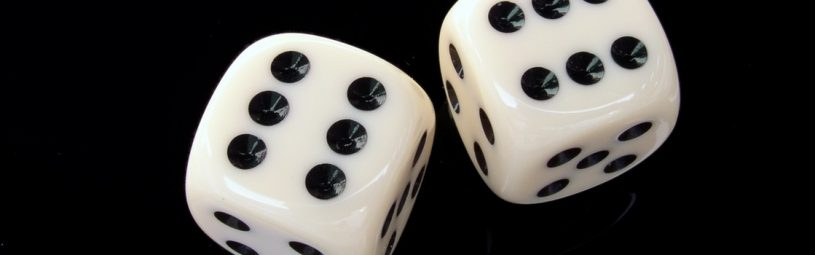# How do you find the odds of something?

June 15th, 2017

Found in both sports and statistics, odds indicate the chances or ‘probability’ of an event taking place. Usually shown as numbers, odds are a ratio relating to the number of ways an event can or can’t happen. They can be expressed as a fraction (eg: 2/1), a decimal (3.0) or a percentage (33%). Now you know what odds are, this post will answer the question “how do you find the odds of something?”

In sports betting, odds show the chances a punter has of winning when they place a bet. The odds will either be ‘against’, ‘odds on’ or ‘evens’.

### Odds against

The chances of a bet losing are greater than its chances of winning. A bet placed at odds against will return a bigger profit than the amount staked – if it wins.

The odds against a bet losing = number of losing outcomes / number of winning outcomes

For example:
A horse race is run with 10 runners of equal ability and form.
Number of losers = 9
Number of winners = 1
The odds against a horse winning the race are 9/1 or 10.0 in decimals.

### Odds on

The opposite of ‘odds against’ so in this case, the chances of a bet winning are greater than its chances of losing. A bet placed at odds on will return a smaller profit than the amount staked, if it wins. It’s still a profit though, as the amount staked on a winning bet is returned.

The odds on a bet winning = number of winning outcomes / number of losing outcomes

For example:
A horse race is run with 10 runners but one of the horses has far better ability and form than the others in the race and should win easily.
Number of winners = 1
Number of losers = 9
The odds on a horse winning the race are 1/9 or 1.11 in decimals.

### Even odds

The chances of a bet winning are equal to the chances of it losing. In other words, the bet has a 50-50 chance of winning. A bet placed at ‘evens’ will return a profit that is equal to the amount staked, if it wins.

The odds against a bet losing = number of losing outcomes / number of winning outcomes
The odds on a bet winning = number of winning outcomes / number of losing outcomes

For even odds, both calculations give the same result.

For example:
A tennis match is played between two players of equal ability and form.
Number of winners = 1
Number of losers = 1
The odds on a player winning the match are 1/1 or 2.0 in decimals.

## Probability in sports betting

When betting on sports, probability is the measure of how likely it is that a bet placed on an event will win or lose. It can be calculated by converting the odds into a percentage (%)

Probability % = 1 / decimal odds

Using the examples set out above:

• The odds against a horse winning the race are 9/1 or 10.0 in decimals.
1 / 10.0 = 0.10 = 10% chance of winning
• The odds on a horse winning the race are 1/9 or 1.11 in decimals.
1 / 1.11 = 0.90 = 90% chance of winning
• The even odds on a player winning the match are 1/1 or 2.0 in decimals.
1 / 2.0 = 0.50 = 50% chance of winning

## Odds and OddsMonkey

So, now you know how to find the odds of something and what the probability of winning is when you place a bet. It’s still risky though, isn’t it?

There is a way of minimising that risk though by ‘matched betting’.

Matched betting isn’t gambling and here at OddsMonkey we have an excellent matched betting tool called the OddsMatcher. It searches online bookmakers and betting exchanges to find the best ‘matched’ bets available and displays them using decimal odds. There are guides and training to get you started.

To find out more about the OddsMatcher and matched betting in general, download our free introduction today.